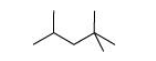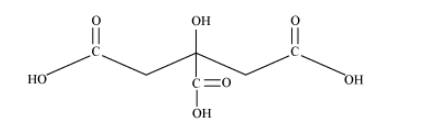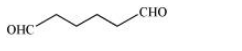# Give condensed and bond line structural formulas and identify the functional group

Question:

Give condensed and bond line structural formulas and identify the functional group(s) present, if any, for :

(a) 2,2,4-Trimethylpentane

(b) 2-Hydroxy-1,2,3-propanetricarboxylic acid

(c) Hexanedial

Solution:

(a) 2, 2, 4–trimethylpentane

Condensed formula:

$\left(\mathrm{CH}_{3}\right)_{2} \mathrm{CHCH}_{2} \mathrm{C}\left(\mathrm{CH}_{3}\right)_{3}$

Bond line formula:(b) 2–hydroxy–1, 2, 3–propanetricarboxylic acid

Condensed Formula:

$(\mathrm{COOH}) \mathrm{CH}_{2} \mathrm{C}(\mathrm{OH})(\mathrm{COOH}) \mathrm{CH}_{2}(\mathrm{COOH})$

Bond line formula:The functional groups present in the given compound are carboxylic acid (–COOH) and alcoholic (–OH) groups.

(c) Hexanedial

Condensed Formula:

$(\mathrm{CHO})\left(\mathrm{CH}_{2}\right)_{4}(\mathrm{CHO})$

Bond line Formula:The functional group present in the given compound is aldehyde

$(-\mathrm{CHO})$